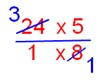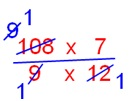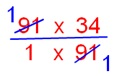# Division of a Whole Number by a Fraction

We will discuss here about division of a whole number by a fraction.

Look at the collection of circles. It has 8 circles.

How
many two’s are there in 8?

How many two’s are there in 8? So we find there are 4 two in
8.

Now what does the following statement mean?

How many halves are there in 8? To find this, let us divide each of the 8 circles into halves.

We find there are 16 halves in 8

So, the answer is 8 ÷ ½ = 16

i.e. = 8 ÷ ½ = 8 × 2/1 = 8 × 2/1 = 16

Therefore, the rules for the division of a whole number by a fractional number is

Whole Number ÷ Fraction = Whole Number × Reciprocal of Fraction.

Hence, we conclude that to divide whole number by a
fractional number, we multiply the whole number by multiplicative inverse of
the fractional number.

Note: Wherever mixed numbers are used first change them into improper fractions.

For Example:

1. 4 ÷ 1/3

[We know, Whole Number × Reciprocal of Fraction]

Reciprocal of Fraction of ‘1/3’ is ‘3’

= 4 × 3

= 12

2. 16 ÷ 2/5

Reciprocal of Fraction of ‘2/5’ is ‘5/2’

= 16 × 5/2

= (16 × 5)/2

= 8 × 5

= 40

3. 24 ÷ 1 3/5

= 24 ÷ (1 × 5 + 3)/5

= 24 ÷ 8/5

Reciprocal of Fraction of ‘8/5’ is ‘5/8’

= 24 × 5/8= 3 × 5

= 15

4. 49 ÷ 7/3

Reciprocal of Fraction of ‘7/3’ is ‘3/7’

= 49 × 3/7= 7 × 3

= 21

5. 11 9/9 ÷ 12/7

= (11 × 9 + 9)/9 ÷ 12/7

= 108/9 ÷ 12/7

Reciprocal of Fraction of ‘12/7’ is ‘7/12’

= 108/9 × 7/12= 7

6. 91 ÷ 91/34

Reciprocal of Fraction of ‘91/34’ is ‘34/91’

= 91 × 34/91= 34